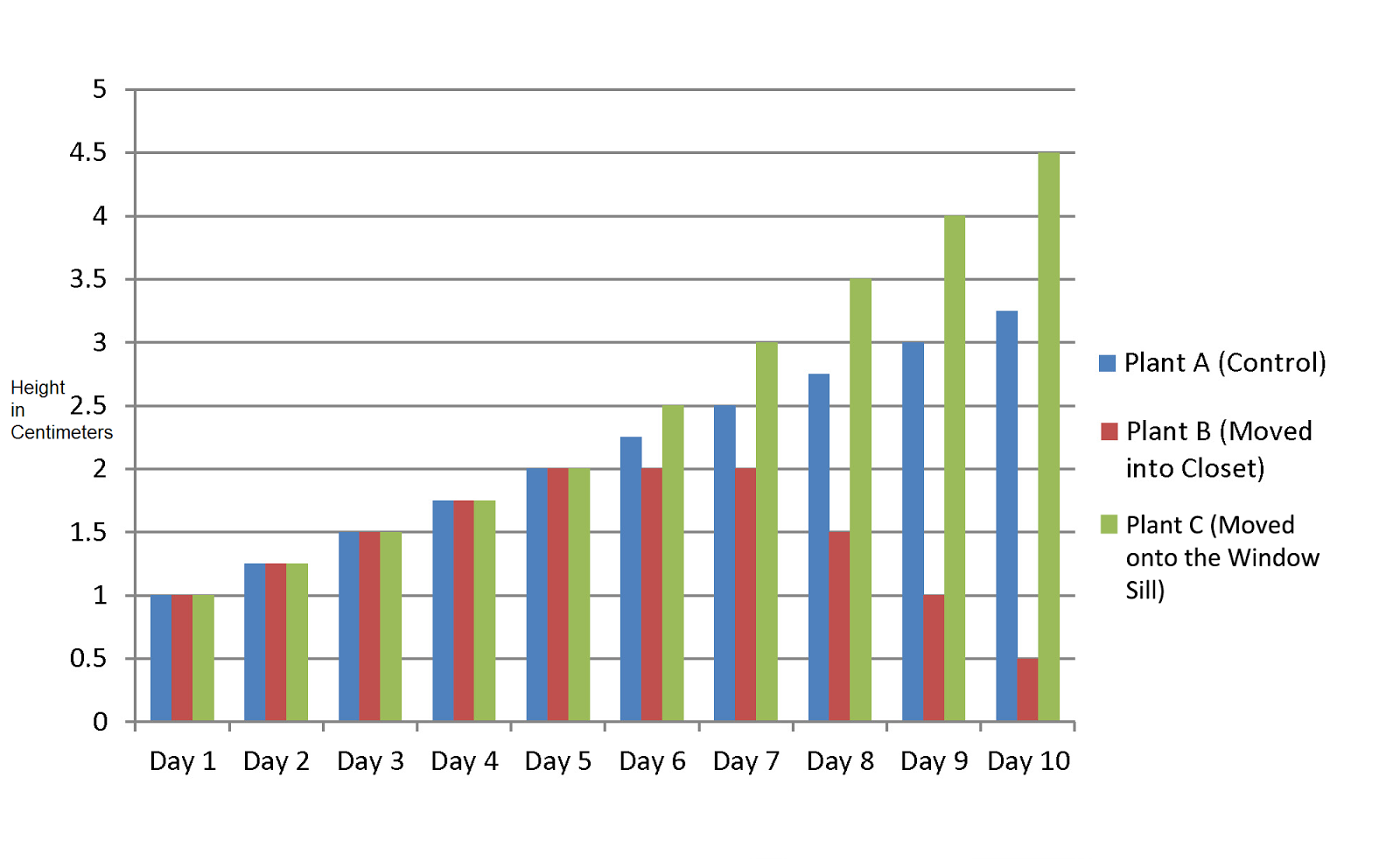Scientific Reasoning Test (HS Level)

This is a short test that is designed to test how well Goodhue students are able to interpret graphs and tables when it comes to experiments. The graph below shows data that was collected by a group of scientists who altered the type/amount of light that a plant was exposed to. The scientists had 10 different posts for each of the test groups and the averages are plotted on the graph. The same soil, plant (pea), soil amount, and water amount was held.

Plant Growth ExperimentThis is a required question
This is a required question
This is a required question
This is a required question
This is a required question
This is a required question